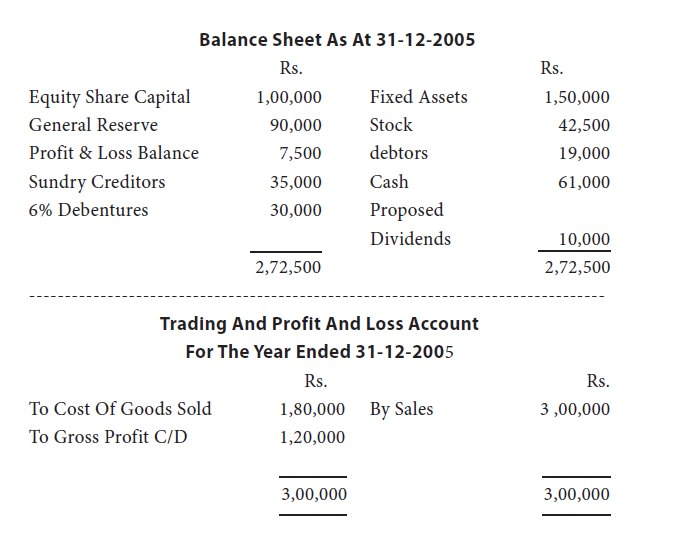Home | ARTS | Accounting For Managers | Ratios From Shareholders’ Point Of View

# Ratios From Shareholders’ Point Of View

Posted On :  27.01.2018 10:37 pm

Shareholders’ Point Of View

1.         Preference dividend cover: this ratio expresses net profit after tax as so many times of preference dividend payable. This is calculated as:

Net Profit After Tax

-------------------------

Preference Dividend

2.         Equity Dividend Cover: this ratio gives information about net profit available to equity shareholders. This ratio expresses profit as number of times of equity dividend payable. This ratio is calculated using the following formula:

Net Profit After Tax – Preference Dividend

-------------------------------------------------

Equity Dividend

3.   Dividend Yield On Equity Shares Or Yield Ratio: this ratio interprets dividend as a percentage of market price per share. It is calculated as:

Dividend Per Share

--------------------------- X 100

Market Price Per Share

4.   Price Earning Ratio: this ratio tells how many times of earnings per share is the market price of the share of a company. The formula to calculate this ratio is:

Market Price Per Share

---------------------------

Earnings Per Share

Illustrations

Illustration 4: the following are the financial statements of yesye limited for the year 2005.To Expenses 1,00,000 By Gross Profit B/D 1,20,000
To Net Profit 20,000
1,20,000 1,20,000

You are required to compute the following:
1) Current Ratio
2) Acid Test Ratio
3) Gross Profit Ratio
4) Debtors’ Turnover Ratio
5) Fixed Assets To Net Tangible Worth
6) Turnover To Fixed Assets
Solution:
click Next to see the calculation
Tags : Accounting For Managers - Ratio Analysis
Last 30 days 242 views